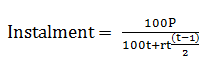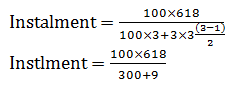# Simple Interest Tricks For IBPS PO 2017: Part 1

Principal: When money is borrowed for a certain time period, called principal or sum.
Amount: The sum of principal and interest is called the amount.
• P – Principal
• r – Rate of interest
• A – Amount
• t – Numbers of years
• S.I. – Simple interest
• C.I. – Compound interest

### 1.#### Example:

What will be the simple interest of Rs. 15400 in 2 years at the rate of 8%?
Solution:= 2464 Rs.

### 2.

If the interest rate and time is in half yearly than for yearly half the time and double the interest rate.

#### Example:

If Mohan deposited 4500 Rs. in bank for 2 years and the rate of interest is 10% half yearly. What will be the simple interest for 2 years?
Solution:S.I.= 1800 Rs.

### 3.

If the interest rate and time is quarterly than for yearly divide the time by 4 and multiply the interest rate by 4.

#### Example:

If Reeta borrowed 5000 Rs. for 3 years and the rate of interest is 3% quarterly. What will be the simple interest for 1 and half year?
Solution:= 900 Rs.

### 4.

If principle, time and rate is given, then find Amount:
P= 5500 Rs., r=5%, t=3 years, A=?
Solution:A= 5500+825
A= 6325 Rs.

### 5.

If the some money becomes x Rs. in 2 years and y Rs. in 3 years then find the principle.
We have to find out the interest of 1 year. Proper explanation in example:

#### Example:

A sum becomes 720 Rs. in 2 years and 780 Rs. in 3 years. Find the principal.
Solution:
Interest in 1 year = 780-720 =60 Rs.
Interest in 2 years = 60×2 = 120 Rs.
Principal = 720-120 = 600 Rs.

### 6.

P= Debt, r= rate of interest, t = time period, Instalment =?#### Example:

What will be annual instalment if the debt is 618 for 3 years at 3% per annum?
Solution:=200 Rs.

### 7.

If the amount is x times of principal and rate of interest and time both are numerically equal. Then:#### Example:

If the amount is (5/4) times of Principal and rate of interest and time both are numerically equal. Then, what is the rate of interest per annum?
Solution:

## Some Examples

### 8.

If some money double itself in 3 years. In how many years it will become 8 times itself at the same rate of interest?

#### Solution:

Let principle = 100 Rs.
It becomes double in 3 years, so now amount = 100×2 = 200 Rs.
Simple interest = 200-100 = 100 Rs.r = 100/3 %
If it becomes 8 times, Interest = 800-100=700t= 21 years

### 9.

If the principal is 500 Rs. the difference between simple interest for 4 years and 6 years is 60 Rs. Find the rate of interest.

#### Solution:

Interest of 1 year =60/2=30 Rs.r= 6 %

### 10.

Rs. 8000 is divided into two parts if one part is invested at 4% and the other at 7% for 1 year, if the total interest from the both investment is 820 Rs. Find each part of investment.

#### Solution:

x×4%×2+(8000-x)×7%×2 = 820
8x+112000-14x= 82000
112000-82000=6x
x=5000 Rs.
1st part = 5000 Rs.
2nd part = 8000-5000 = 3000 Rs.